# AP Calculus BC Practice Test 17

### Test Information9 questions18 minutes

Calculator Disallowed

1. Use the table below, which shows the values of differentiable functions f and g.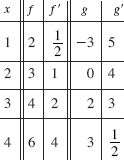If H(x) = f -1(x), then H (3) equals

2.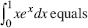3. The graph of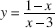is concave upward when

4. As an ice block melts, the rate at which its mass, M, decreases is directly proportional to the square root of the mass. Which equationdescribes this relationship?

5. The length of the curve y = 2x3/2 between x = 0 and x = 1 is equal to

6. If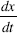= kx, and if x = 2 when t = 0 and x = 6 when t = 1, then k equals

7. If y = x2 ln x (x > 0), then y is equal to

8. A particle moves along the curve given parametrically by x = tan t and y = 2 sin t. At the instant when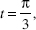the particle's speed equals

9. Suppose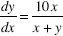and y = 2 when x = 0. Use Euler's method with two steps to estimate y at x = 1.